# When a large cube is placed in a tub of water, it floats with 0.5 m...

When a large cube is placed in a tub of water, it floats with 0.5 m of it submerged. When the same cube is placed in anunknown liquid, 3.5 m of it is submerged. What is the density of the unknown liquid (in kg/m3)?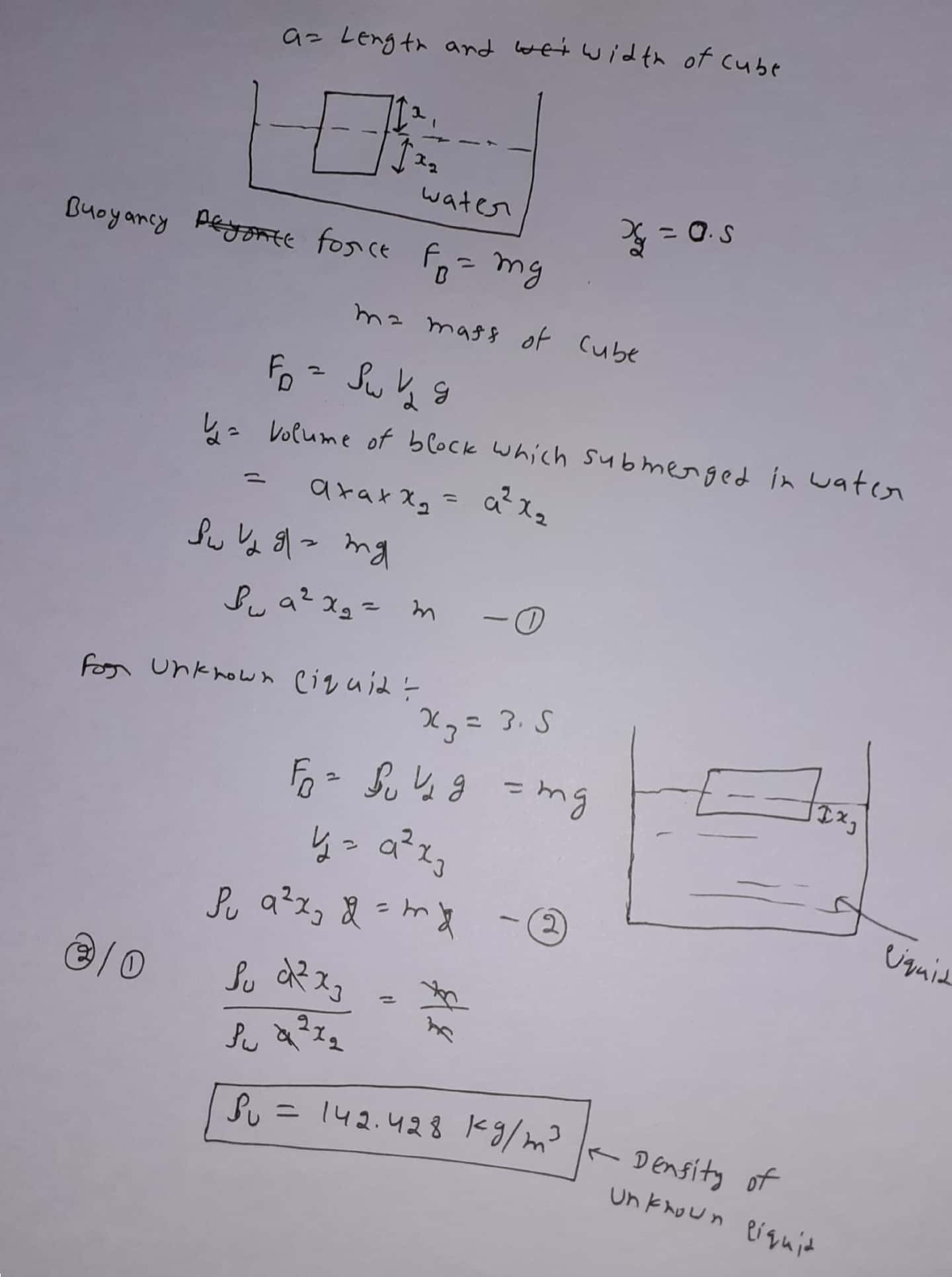##### Add Answer of: When a large cube is placed in a tub of water, it floats with 0.5 m...
Similar Homework Help Questions
• ### A cube is placed in a tub of water and floats with 2.50 m of the...

A cube is placed in a tub of water and floats with 2.50 m of the cube submerged. An identical cube is placed in a tub of an unknown liquid, and floats with 1.50 m of it submerged. What is the density of the unknown liquid in kg/m3?

• ### 6.) A plastic cube is placed in a cup of pure water and it floats with 28.0 % of its volume above the surface. The...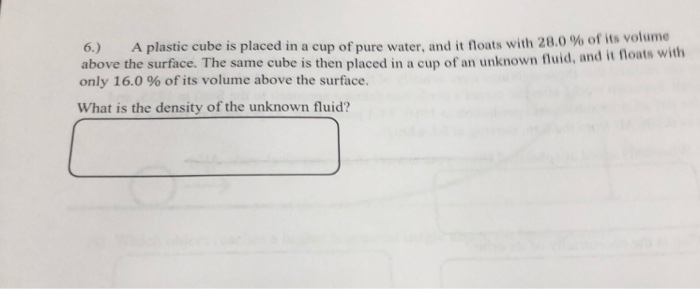6.) A plastic cube is placed in a cup of pure water and it floats with 28.0 % of its volume above the surface. The same cube is then placed in a cup of an unknown fluid, and it floats with only 16.0 % of its volume above the surface. What is the density of the unknown fluid?

• ### A wooden cube with edge length 0.843 m has density 6.00×102 kg/m3. When the cube is...

A wooden cube with edge length 0.843 m has density 6.00×102 kg/m3. When the cube is placed in fresh water, to what depth will it be submerged? ans ) 0.506 m if you did not get it dont try to answer

• ### Fluids

When a block of volume 1.00 × 10–3 m3 is hung from a spring scale and is completely submerged in water, the scale reads 10.0 N. When thesame block is then placed in an unknown liquid, unattached to a scale, it floats with 2/3 of its volume submerged in the unknown fluid. The density of water is1.00 × 103 kg/m3. Determine the density of the unknown liquid.

• ### 5)A plastic sphere floats in water with 70.0% of its volume submerged. This same sphere floats in glycerin with 55.6% o...

5)A plastic sphere floats in water with 70.0% of its volume submerged. This same sphere floats in glycerin with 55.6% of its volume submerged. (a) Determine the density of the glycerin. kg/m3 (b) Determine the density of the sphere. kg/m3

• ### A plastic sphere floats in water with 35.0% of its volume submerged. This same sphere floats...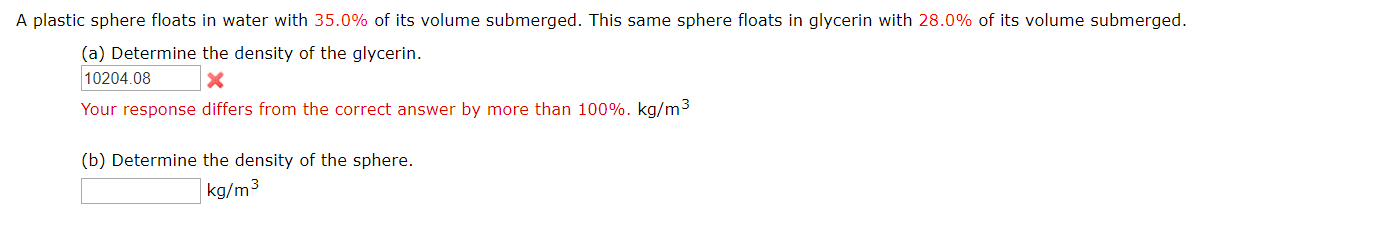A plastic sphere floats in water with 35.0% of its volume submerged. This same sphere floats in glycerin with 28.0% of its volume submerged. (a) Determine the density of the glycerin 10204.08 X Your response differs from the correct answer by more than 100%. kg/m3 (b) Determine the density of the sphere. kg/m3

• ### 4) A) A 10 kg cube of lucite (plastic, get the density from the web) floats...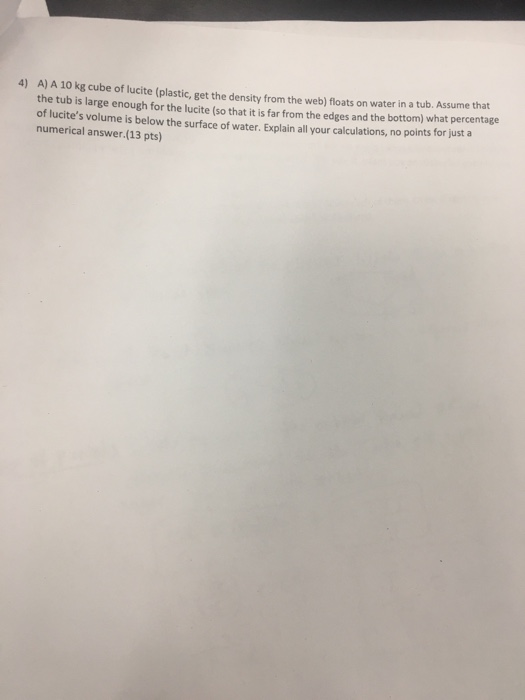4) A) A 10 kg cube of lucite (plastic, get the density from the web) floats on water in a tub. Assume that the tub is large enough for the lucite (so that it is far from the edges and the bottom) what percentage of lucite's volume is below the surface of water. Explain all your calculations, no points for just a numerical answer. (13 pts)

• ### A wooden cylinder 30.0 cm high floats vertically in a tub of water. The top of...

A wooden cylinder 30.0 cm high floats vertically in a tub of water. The top of the cylinder is 14.1 cm above the surface of the liquid. What is the density of the wood?

• ### A plastic sphere floats in water with 45.0% of its volume submerged. This same sphere floats...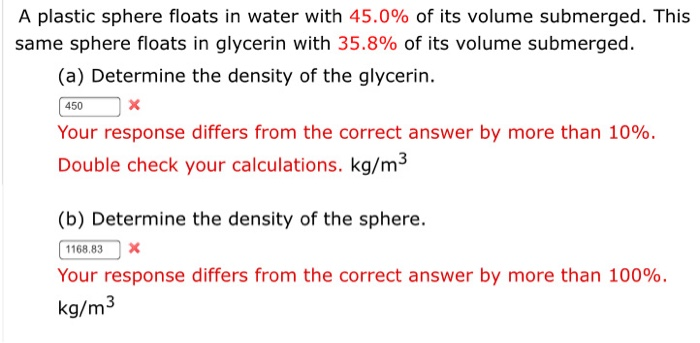A plastic sphere floats in water with 45.0% of its volume submerged. This same sphere floats in glycerin with 35.8% of its volume submerged (a) Determine the density of the glycerin. 450 Your response differs from the correct answer by more than 10%. Double check your calculations. kg/m3 (b) Determine the density of the sphere. 1168.83 Your response differs from the correct answer by more than 100%. kg/m3

• ### A cube of unknown material which is 1 m on a side is observed to float in fresh water with 35%...

A cube of unknown material which is 1 m on a side is observed to float in fresh water with 35% of the cube exposed above the water line.(a) What is the density of the material?kg/m3(b) If the same cube is taken to the dead sea (? = 1240 kg/m3), how much of the cube (in percent) is above the water line?%

Free Homework App